# The Power Rule for Derivatives: What is the Power Rule?

Riley Kench, Kimberlee Davison, Kathryn Boddie
• Author
Riley Kench

Riley has tutored collegiate mathematics for seven years. They have a Master of Arts degree in Mathematics from Central Michigan University and a Bachelor of Science degree in Mathematics from Central Michigan University.

• Instructor
Kimberlee Davison

Kim has a Ph.D. in Education and has taught math courses at four colleges, in addition to teaching math to K-12 students in a variety of settings.

• Expert Contributor
Kathryn Boddie

Kathryn has taught high school or university mathematics for over 10 years. She has a Ph.D. in Applied Mathematics from the University of Wisconsin-Milwaukee, an M.S. in Mathematics from Florida State University, and a B.S. in Mathematics from the University of Wisconsin-Madison.

In this lesson, learn the power rule for the derivative of exponents. Moreover, learn to understand how to apply the power rule of derivatives for various cases including negative powers. Updated: 09/30/2021

Show

## What is the Power Rule?

In calculus, what is the power rule? The power rule, which is also called the exponent rule, is a rule that tells the derivative of a power function of the form {eq}f(x)=ax^n {/eq} for {eq}a, x \in \mathbb{R} {/eq} and {eq}a, n \neq 0 {/eq}. Now, a power function is any function of the form above; that is, it is a function of the form of x raised to a fixed power n and multiplied by some coefficient a. Here are some examples of power functions:

• {eq}f(x)=\pi*x^2 {/eq}
• {eq}f(x)=-x^5 {/eq}
• {eq}f(x)=3x^{-1} {/eq}

Notice that the powers of x in these examples can be both positive and negative and that the coefficients can also be both positive and negative.

Here is the graph of {eq}y=x^2 {/eq}. All power functions of positive even power ({eq}x^{2n} {/eq}) will have this general shape: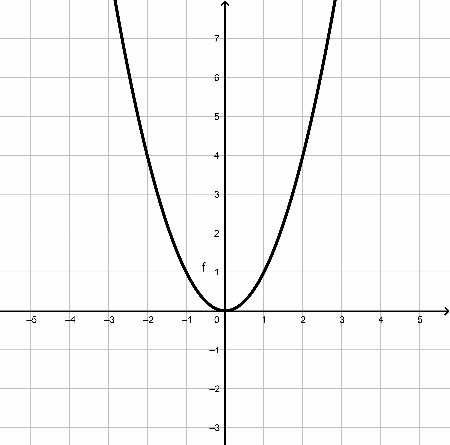Similarly, power functions of positive odd power will have the same general shape. Below is the graph of {eq}y=x^3 {/eq} which will show the typical shape of a positive odd-powered power function ({eq}x^{2n+1} {/eq}):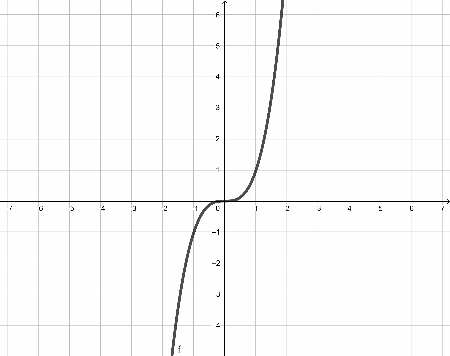Although power functions can look differently, they will always be of the forms above depending on if they are odd-powered or even-powered power functions.

Now, although power functions can appear differently when graphed, differentiating them involves a simple rule called the power rule. This rule will give the derivative for any power function (and later on, any sum of power functions as well as power functions of negative exponent).

The power rule formula for a fundamental power function is:

• {eq}\frac{d}{dx}x^n=nx^{n-1} {/eq}

Simply put, if given a basic power function of the form {eq}x^n {/eq}, its derivative is given by bringing down the power of x (n) and multiplying it to the function, and then subtracting one from the exponent of x. If the power function has a coefficient, then its derivative will be:

• {eq}\frac{d}{dx}ax^n=nax^{n-1} {/eq}. This is also a form of the constant multiple rule which states that {eq}\frac{d}{dx}(cf(x))=c*\frac{d}{dx}f(x)=cf'(x) {/eq}.

Here is the proof of the power rule for {eq}x^n {/eq}:

#### Proof:

• Start with the limit definition of the derivative: {eq}\frac{d}{dx}f(x)=\lim_{h \rightarrow 0}\frac{f(x+h)-f(x)}{h} {/eq}.
• Now, substitute the power function for f(x): {eq}\lim_{h \rightarrow 0}\frac{(x+h)^n-x^n}{h} {/eq}.
• Now, expand the {eq}(x+h)^n {/eq} term so the limit becomes: {eq}\lim_{h \rightarrow 0}\frac{(x+h)(x+h)...(x+h)-x^n}{h} {/eq}. In this limit, there are n copies of (x+h) being multiplied.
• In this product of n-terms, the leading term will be {eq}x^n {/eq} and the next term following that one will be {eq}nhx^{n-1} {/eq} followed by many terms all containing powers of h.
• Since the limit is subtracting {eq}x^n {/eq}, the limit then becomes: {eq}\lim_{h \rightarrow 0}\frac{nhx^{n-1}+...}{h} {/eq}. In this limit, the ellipsis indicates the terms following the initial term that all contain higher powers of h.
• Dividing all the terms by h gives: {eq}\lim_{h \rightarrow 0}nx^{n-1}+... {/eq}
• Finally, as {eq}h \rightarrow 0 {/eq}, the terms indicated by the ellipsis become zero which leaves the final term of {eq}nx^{n-1} {/eq}.
• Therefore, the derivative becomes: {eq}\frac{d}{dx}x^n=nx^{n-1} \square {/eq}

This shows that the power rule for the derivative of a power function will work for any power function. Next are some examples of the power rule.

### Power Rule Examples

Here are some examples of using the power rule to find the derivative of a power function (note that {eq}f'(x) {/eq} denotes the derivative of f(x).):

• Let {eq}f(x)=2x^2 {/eq}. Then {eq}f'(x)=(2)(2)x^{2-1}=4x^1=4x {/eq}.
• Let {eq}f(x)=-x^{3} {/eq}. Then {eq}f'(x)=(3)(-1)x^{3-1}=-3x^{2} {/eq}.
• Consider {eq}f(x)=1000x^1 {/eq}. Then {eq}f'(x)=(1)(1000)x^{1-1}=1000x^{0}=1000 {/eq}.
• Given {eq}f(x)=3x^7 {/eq} then its derivative would be {eq}f'(x)=(7)(3)x^{7-1}=21x^6 {/eq}.
• Let {eq}f(x)=6x^{5} {/eq}. Its derivative would then be {eq}f'(x)=(5)(6)x^{5-1}=30x^{4} {/eq}.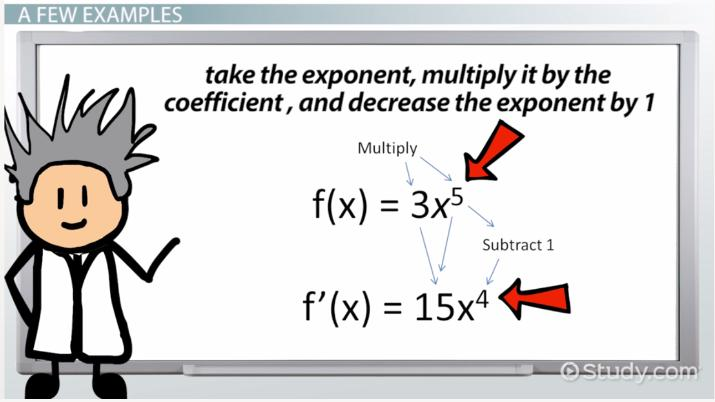An error occurred trying to load this video.

Try refreshing the page, or contact customer support.

Coming up next: Trigonometry: Sine and Cosine

### You're on a roll. Keep up the good work!

Replay
Your next lesson will play in 10 seconds
• 0:00 What Is the Power Rule?
• 0:26 A Few Examples
• 2:02 Working with…
• 3:35 Lesson Summary
Save Save

Want to watch this again later?

Timeline
Autoplay
Autoplay
Speed Speed

## Derivatives of Power Functions

In the preceding sections, only basic power functions were considered. But, what if instead of a single power function, there is a sum or difference of different power functions? This is actually called a polynomial and has the form {eq}f(x)=a_{n}x^n+a_{n-1}x^{n-1}+ ... + a_{1}x^{1}+a_{0} {/eq} where the {eq}a_{i} {/eq} are constant coefficients.

Now, using the power rule, constant multiple rule, and the sum rule, the derivatives of polynomial functions like the ones described above can be found. Moreover, the sum rule states that that states that: {eq}\frac{d}{dx}(f(x)+g(x))=\frac{d}{dx}f(x)+\frac{d}{dx}g(x)=f'(x)+g'(x) {/eq}. This essentially says that the derivative of a sum is the sum of the derivatives.

Therefore, in order to differentiate polynomials of power functions, simply differentiate each term of the polynomial function independently and then add them together. Here is an example involving the general form of the polynomial:

• {eq}\frac{d}{dx}(f(x)=a_{n}x^n+a_{n-1}x^{n-1}+ ... + a_{1}x^{1}+a_{0})= {/eq}
• {eq}\frac{d}{dx}a_{n}x^n+\frac{d}{dx}a_{n-1}x^{n-1}+ ... + \frac{d}{dx}a_{1}x^{1}+\frac{d}{dx}a_{0}= {/eq}
• {eq}na_{n}x^{n-1}+(n-1)a_{n-1}x^{n-2}+ ... + (1)a_{1}x^{0}+(0)a_{0}= {/eq}
• {eq}na_{n}x^{n-1}+(n-1)a_{n-1}x^{n-2}+ ... + a_{1} {/eq}

To unlock this lesson you must be a Study.com Member.

• Activities
• FAQs

## Why Use the Power Rule When There Is Already a Definition?

How do we know the power rule is equivalent to the derivative given by using the limit definition of the derivative? Recall that the definition of the derivative of a function f(x) is given by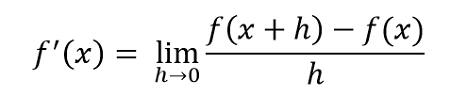Why should we memorize another rule rather than just using the definition of the derivative? The answer to this question is that the rule is MUCH quicker and easier to work with.

## Examples

Find the derivative of the following functions using the definition of the derivative and then by using the power rule to show that both results are equal.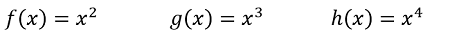## Solutions

1. For f(x), using the definition, we get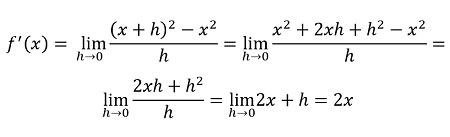Using the power rule instead, we get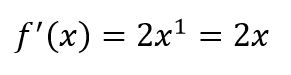The answers agree and the power rule was much quicker!

2. For g(x), using the definition, we get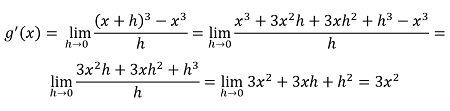Using the power rule instead, we get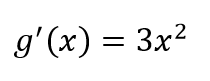The answers agree again, and the power rule was much simpler to use.

3. For h(x), using the definition we get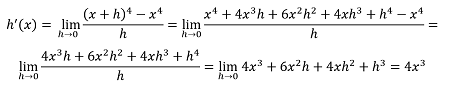Using the power rule instead, we get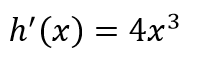The answers agree again and the power rule was, once again, much easier to use.

## Why Does This Work?

Although the examples above are not a proof, they likely convinced you that the power rule works. The actual proof is done the same way - using the limit definition of the derivative for the function x to the nth power. However, if you do not know the Binomial Theorem, it can be tricky to write out. The idea is that when you expand (x+h) to any power, you get a term with just x to a power, and then every single other term has an h in it. The second term in the expansion is always equal to nx^(n-1) h , and all the other terms with h's in them have h to a power higher than 1. The first term of the expansion, x^n, disappears when combining f(x+h) - f(x) in the numerator of our definition. Then, all other terms have an h cancel out with the h in the denominator - your first term of what is left is now nx^(n-1) and all other terms still have at least one h multiplied in them. So, when h goes to 0, all terms vanish except for the term given by the power rule: nx^(n-1). If that was hard to follow - try reading through it again while looking at the examples done above.

#### How does the power rule work?

The power rules works on power functions of the form f(x)=ax^n. The rule finds the derivative by first bringing the power of x (n) down and multiplying it to the function and then subtracting one from the power. The derivative is then f'(x)=nax^(n-1).

#### How do you find the derivative when the variable is in the exponent?

If the variable is in the exponent, the function has the form f(x)=a(b)^x with a,b real numbers. The power rule described in this lesson only works for power functions of the form f(x)=ax^n where a, n are real numbers and both nonzero. For the exponential function f(x)=a(b)^x, the derivative is given by f'(x)=a*ln(b)*(b)^x but it is not part of this lesson.

#### What is the power rule in exponents?

The power rule for the derivative of a power function is (ax^n)'=nax^(n-1). That is, if a function f(x)=ax^n is given with a, n both real numbers and nonzero, then its derivative is given by f'(x)=nax^(n-1) (bring down the power and multiply it to the function and then subtract one from the power).

### Register to view this lesson

Are you a student or a teacher?